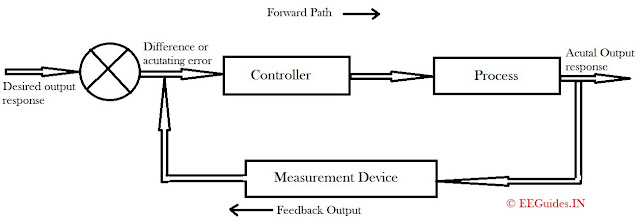# Closed Loop Control SystemPhoto Credit- www.hitecproducts.no

A closed loop control system utilizes an additional measure of the actual output to compare the actual output with the desired output response. The measure of the output is called the feedback signal. A feedback control system is a control system that tends to maintain a relationship of one system variable to another by comparing function of these variables and using the difference as means of control. A the system is becoming more complex, the interrelationship of many controlled variables may be considered in the control scheme. An example of closed loop control system is a person steering an auto mobile by looking at the auto's location on the road and making the appropriate adjustments.Fig.Closed Loop Feedback System

Following figure shows an example of block diagram of a closed loop system. With reference to this figure, the terminology used in block diagram of control system is given below:Terminology used in block diagram
From the figure it can be clearly seen that the output C(s) is fed back to the summing point where it is compared with the reference input R(s). The output of the block C(s) is obtain by multiplying the transfer function G(s) by the input to the block E(s).Terminology Used In Closed Loop System
When the output is fed back to the summing point for comparison with the input, it is important to convert the form of the output signal to that of the input, This is done by element H(s). H(s) is known as feedback element. It is generally, a sensor which modifies the output before it is compared with the input. When the output of H(s) that is B(s) is compared with the reference input signal R(s), an actuating error signal E(s) is generated. In the present example the feedback signal that is fed back to the summing point for comparison with the input R(s) is B(s)=H(s)C(s)

Previous Post
Next Post